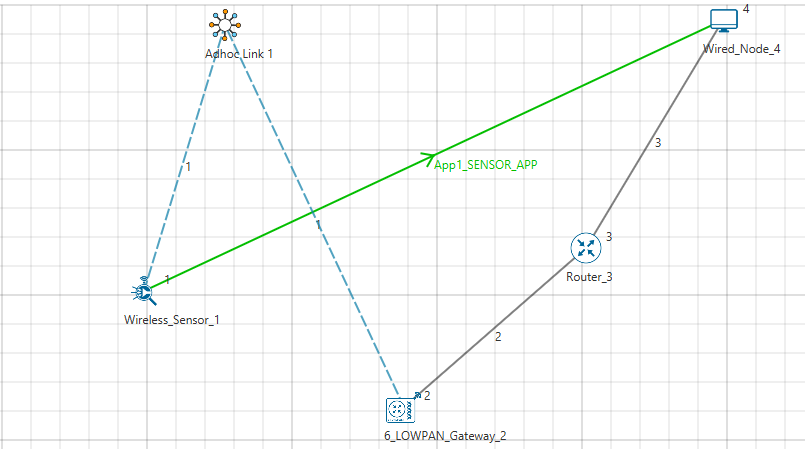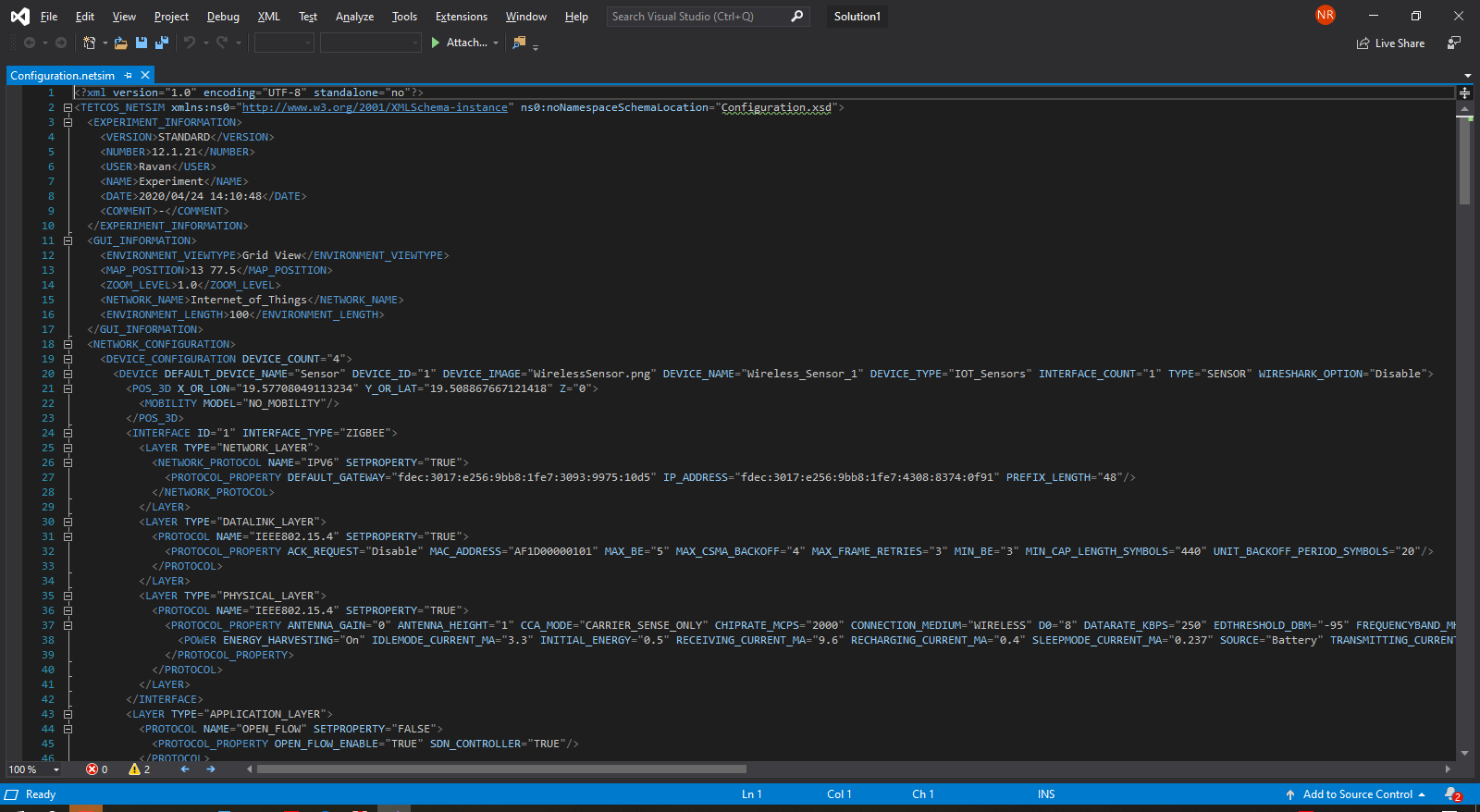Applicable Versions Netsim Academic Netsim Standard Netsim Pro

Any network scenario created in NetSim GUI is converted into an XML based Configuration.netsim file. The various sections of the Configuration file are explained in Section 12.3 of the NetSim User Manual. Similarly, the Configuration file can act as an input to NetSim GUI to load a saved network scenario. Any configuration that can be done in the GUI can also be done via Configuration.netsim file.

As an example you could refer to the following knowledge base article link which demonstrates the addition of a wireless node in MANET network scenario via a Configuration file:

Following is an example associated with the IoT network scenario in NetSim:

• In the NetSim IoT module click and drop a sensor, LoWPAN gateway, a router, and a wired node. Establish connections and configure an application.• Save the network scenario to obtain a dummy configuration file to obtain the outline of the IoT Configuration file.• Further, since the configuration file is organized into different sections each enclosed within tags, you could individually generate different sections of the Configuration file via scripts and combine them to form the complete Configuration file.

For example,

Device Configuration:

In the case of Device configuration, let's assume that all devices are initially going to have similar properties, except unique properties such as DEVICE_IDDEVICE_NAME, IP_ADDRESS, MAC_ADDRESS, X_OR_LON, Y_OR_LAT, etc.

Then you could write a script that uses the general structure of the Device Configuration as shown below and writes a file that contains a number of devices as per your requirement:

<DEVICE_CONFIGURATION DEVICE_COUNT="4">

<DEVICE DEFAULT_DEVICE_NAME="Sensor" DEVICE_ID="1" DEVICE_IMAGE="WirelessSensor.png" DEVICE_NAME="Wireless_Sensor_1" DEVICE_TYPE="IOT_Sensors" INTERFACE_COUNT="1" TYPE="SENSOR" WIRESHARK_OPTION="Disable">

<POS_3D X_OR_LON="19.57708049113234" Y_OR_LAT="19.508867667121418" Z="0">

<MOBILITY MODEL="NO_MOBILITY"/>

</POS_3D>

<INTERFACE ID="1" INTERFACE_TYPE="ZIGBEE">

<LAYER TYPE="NETWORK_LAYER">

<NETWORK_PROTOCOL NAME="IPV6" SETPROPERTY="TRUE">

</NETWORK_PROTOCOL>

</LAYER>

<PROTOCOL NAME="IEEE802.15.4" SETPROPERTY="TRUE">

<PROTOCOL_PROPERTY ACK_REQUEST="Disable" MAC_ADDRESS="AF1D00000101" MAX_BE="5" MAX_CSMA_BACKOFF="4" MAX_FRAME_RETRIES="3" MIN_BE="3" MIN_CAP_LENGTH_SYMBOLS="440" UNIT_BACKOFF_PERIOD_SYMBOLS="20"/>

</PROTOCOL>

</LAYER>

<LAYER TYPE="PHYSICAL_LAYER">

<PROTOCOL NAME="IEEE802.15.4" SETPROPERTY="TRUE">

<PROTOCOL_PROPERTY ANTENNA_GAIN="0" ANTENNA_HEIGHT="1" CCA_MODE="CARRIER_SENSE_ONLY" CHIPRATE_MCPS="2000" CONNECTION_MEDIUM="WIRELESS" D0="8" DATARATE_KBPS="250" EDTHRESHOLD_DBM="-95" FREQUENCYBAND_MHZ="2400" MIN_LIFS_PERIOD_SYMBOLS="40" MIN_SIFS_PERIOD_SYMBOLS="12" MODULATION_TECHNIQUE="O-QPSK" PHY_SHRDURATION="3" PHY_SYMBOLPEROCTET="5.3" RECEIVER_SENSITIVITY_DBM="-85" SYMBOLRATE_KSYMBOLSPS="62.5" TURNAROUNDTIME_SYMBOLS="12" TX_POWER="1" UNIT_BACKOFFTIME_SYMBOLS="20">

<POWER ENERGY_HARVESTING="On" IDLEMODE_CURRENT_MA="3.3" INITIAL_ENERGY="0.5" RECEIVING_CURRENT_MA="9.6" RECHARGING_CURRENT_MA="0.4" SLEEPMODE_CURRENT_MA="0.237" SOURCE="Battery" TRANSMITTING_CURRENT_MA="8.8" VOLTAGE_V="3.6"/>

</PROTOCOL_PROPERTY>

</PROTOCOL>

</LAYER>

</INTERFACE>

<LAYER TYPE="APPLICATION_LAYER">

<PROTOCOL NAME="OPEN_FLOW" SETPROPERTY="FALSE">

<PROTOCOL_PROPERTY OPEN_FLOW_ENABLE="TRUE" SDN_CONTROLLER="TRUE"/>

</PROTOCOL>

</LAYER>

<LAYER TYPE="TRANSPORT_LAYER">

<PROTOCOL NAME="TCP" SETPROPERTY="TRUE">

<PROTOCOL_PROPERTY ACKNOWLEDGEMENT_TYPE="Undelayed" CONGESTION_ALGORITHM="NEW_RENO" INITIAL_SSTHRESH="65535" MAX_SYN_RETRIES="5" SACK_PERMITTED="FALSE" SELECTIVE_ACK="FALSE" TCP_MSS="1460" TIMESTAMP_OPTION="FALSE" TIME_WAIT_TIMER="120" WINDOW_SCALING="FALSE"/>

</PROTOCOL>

<PROTOCOL NAME="UDP" SETPROPERTY="TRUE">

<PROTOCOL_PROPERTY/>

</PROTOCOL>

</LAYER>

<LAYER TYPE="NETWORK_LAYER">

<ROUTING_PROTOCOL NAME="AODV" SETPROPERTY="TRUE">

<PROTOCOL_PROPERTY/>

</ROUTING_PROTOCOL>

</LAYER>

</DEVICE>

The Device properties can then be copied to the dummy configuration file.

Application Configuration:

Similarly, in case of application configuration, let's assume a case where the APPLICATION_TYPE, PACKET_SIZE, INTER_ARRIVAL_TIME, etc constant, with either or both SOURCE_ID and DESTINATION_ID varying for each application, then you could write a script which uses the general structure of the application type as shown below and writes a file which contains a number of applications as per your requirement:

<APPLICATION_CONFIGURATION COUNT="1">

<APPLICATION APPLICATION_COLOR="0x03be00ff" APPLICATION_METHOD="UNICAST" APPLICATION_TYPE="SENSOR_APP" APPLICATION_WIDTH="2.0" DESTINATION_COUNT="1" DESTINATION_ID="4" ENCRYPTION="NONE" END_TIME="100000" ID="1" NAME="App1_SENSOR_APP" PRIORITY="Low" PROTOCOL="NONE" QOS="BE" RANDOM_STARTUP="FALSE" SHOW_LINE="Show line" SOURCE_COUNT="1" SOURCE_ID="1" START_TIME="0" TRANSPORT_PROTOCOL="UDP">

<PACKET_SIZE DISTRIBUTION="CONSTANT" VALUE="50"/>

<INTER_ARRIVAL_TIME DISTRIBUTION="CONSTANT" VALUE="1000000"/>

</APPLICATION>

</APPLICATION_CONFIGURATION>

The application properties can then be copied to the dummy configuration file.

After performing changes to Device and application configuration and suitably editing the other sections such as DEVICE_COUNT, CONNECTION link properties, COUNT of applications etc you can open the Configuration in NetSim GUI using Import Configuration file option under Open simulation.Related article:

how-to-access-and-modify-the-config-file-of-the-network-scenario-created-in-netsim-gui-